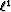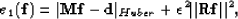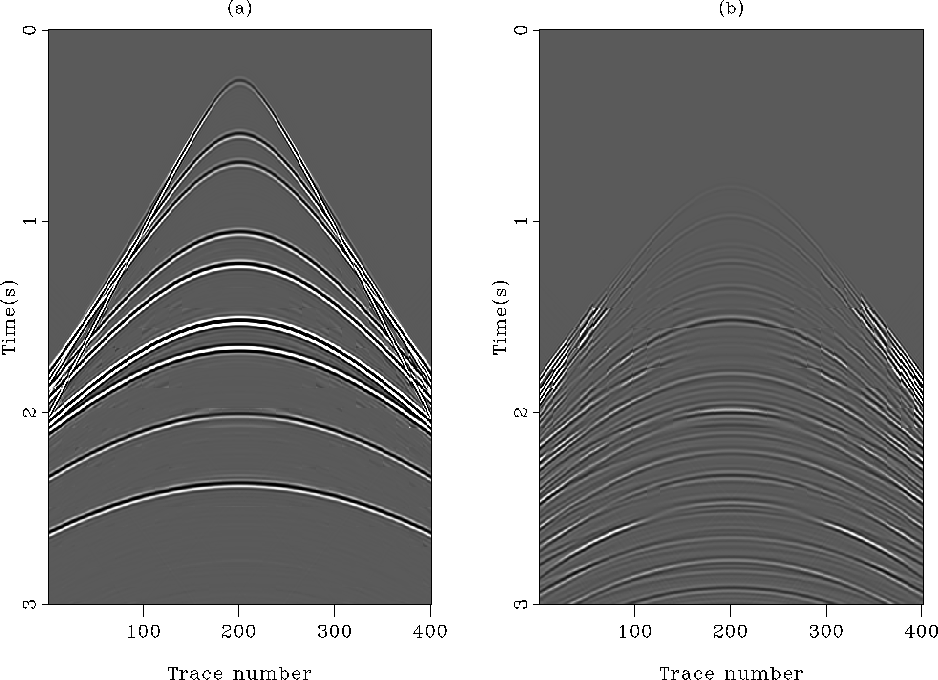Next: Adaptive subtraction results Up: 2-D data example: attenuation Previous: The synthetic data

### Adaptive filtering with non-stationary helical filters

To handle the inherent non-stationarity of seismic data, I estimate a bank of non-stationary filters using helical boundary conditions Claerbout (1998); Mersereau and Dudgeon (1974). This approach has been successfully utilized by Rickett et al. (2001) to attenuate surface-related multiples. As described before [equation ()], I use the Huber norm to approximate thenorm and a standard conjugate-gradient solver with thenorm. The filter coefficients vary smoothly across the output space by introducing a regularization term inside equation () Crawley (2000); Rickett et al. (2001). The misfit function to minimize becomes(13)interl2
Figure 7
(a) The estimated primaries with thenorm. (b) The estimated internal multiples with thenorm. Ideally, (b) should look like Figureb, but it does not.interl1
Figure 8
(a) The estimated primaries with thenorm. (b) The estimated internal multiples with thenorm. Beside some edge-effects, (b) resembles closely Figureb. The adaptive subtraction worked very well.whereis the unknown vector of filter coefficients for the non-stationary matching filters andis a regularization operator. The Helix derivative Claerbout (1998) is chosen for.In the following results, the non-stationary filters are 1-D. The same number of coefficients per filter are estimated with bothandnorms.Next: Adaptive subtraction results Up: 2-D data example: attenuation Previous: The synthetic data
Stanford Exploration Project
5/5/2005